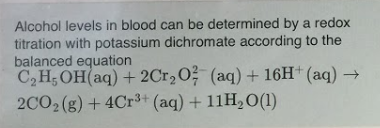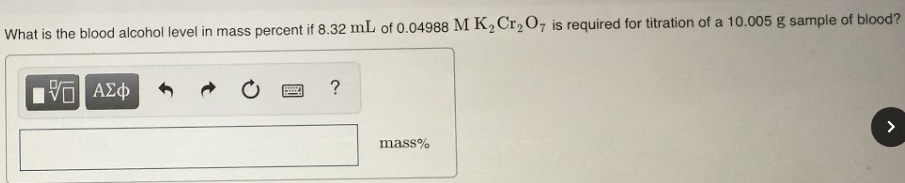# Alcohol levels on blood can be determined by a redox titration with potassium dichromate according to the balanced equation. C2H5OH(aq) + 2Cr2O7(aq) + 16H^+(aq) → 2CO2(g) + 4Cr^3+(aq) + 11H2O(l) What is the blood alcohol level in mass percent if 8.32 mL of 0.04988 M K2Cr2O7 is required for titration of a 10.005 g sample of blood?